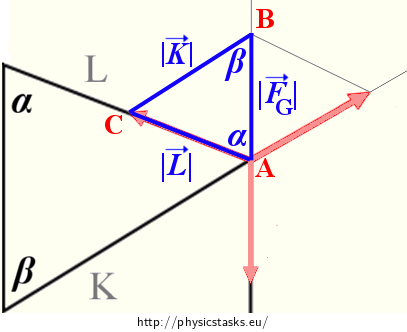## Decomposition of Forces

Weight FG is acting on a body attached to two rods K and L (see Fig. 1).Figure 1

Express the magnitudes of forces exerted on the rods K and L.

• #### Hint 1

Which forces act at the contact point of the rods and suspension cord and in which direction? Draw them in the picture. What is the resultant force?

• #### Hint 2

Draw the resultant of forces K and L in Figure 2. Its magnitude is known. Mark angles α and β in the arisen parallelogram of forces. Select one of the triangles in which you know the magnitude of all three angles and one side. Can we apply some mathematical rule to this triangle (which is generally not rectangular) and express the length of two remaining sides which correspond to the magnitudes of forces K and L?

• #### Hint 3

Write the sine rule for triangle ABC and express the magnitude of forces K and L from it.

• #### Overall Solution

The following forces are present at the contact point of the rods and suspension cord (see Fig. 2):

• pushing force K in the direction of rod K,
• pulling force L in the direction of rod L,
• weight of the body in the vertical direction G = FG.Since the suspension cord is at rest with respect to the supporting structure, the resultant force must equal to zero:

$\vec{F}_G\,+\,\vec{K}\,+\,\vec{L}\,=\,\vec{o}.$

Forces FG, K and L form the triangle ABC, shown in Figure 3.Since we know the magnitude of all its angles and side AB of the triangle, we can apply the sine rule on it:

$\frac{K}{\sin{\alpha}}\,=\,\frac{L}{\sin{\beta}}\,=\,\frac{F_G}{\sin({\pi\,-\,(\alpha\,+\,\beta))}}.\tag{1}$

Sine function has the following property:

$\sin{(\pi\,-\,(\alpha\,+\,\beta))}\,=\,\sin(\alpha\,+\,\beta).\tag{2}$

Thus, combining relations (1) and (2), we obtain:

$K\,=\,\frac{F_G\sin{\alpha}}{\sin(\alpha\,+\,\beta)},$ $L\,=\,\frac{F_G\sin{\beta}}{\sin(\alpha\,+\,\beta)}.$
$K\,=\,\frac{F_G\sin{\alpha}}{\sin(\alpha\,+\,\beta)},$ $L\,=\,\frac{F_G\sin{\beta}}{\sin(\alpha\,+\,\beta)}.$